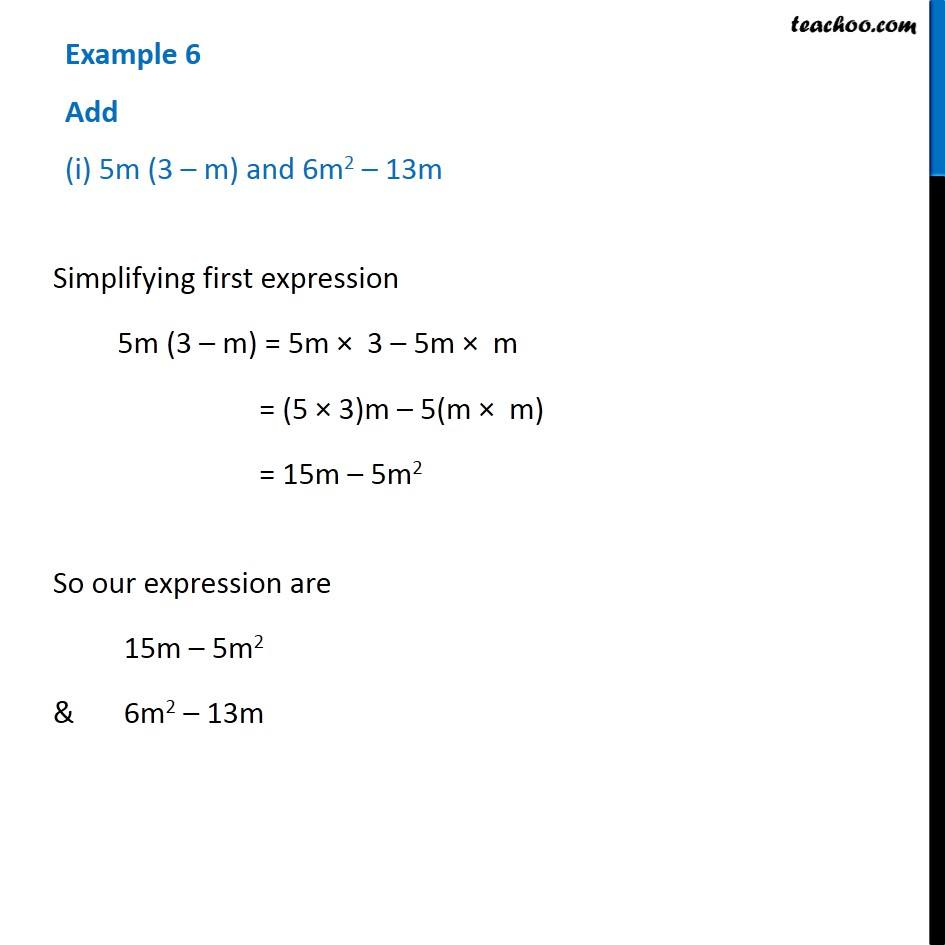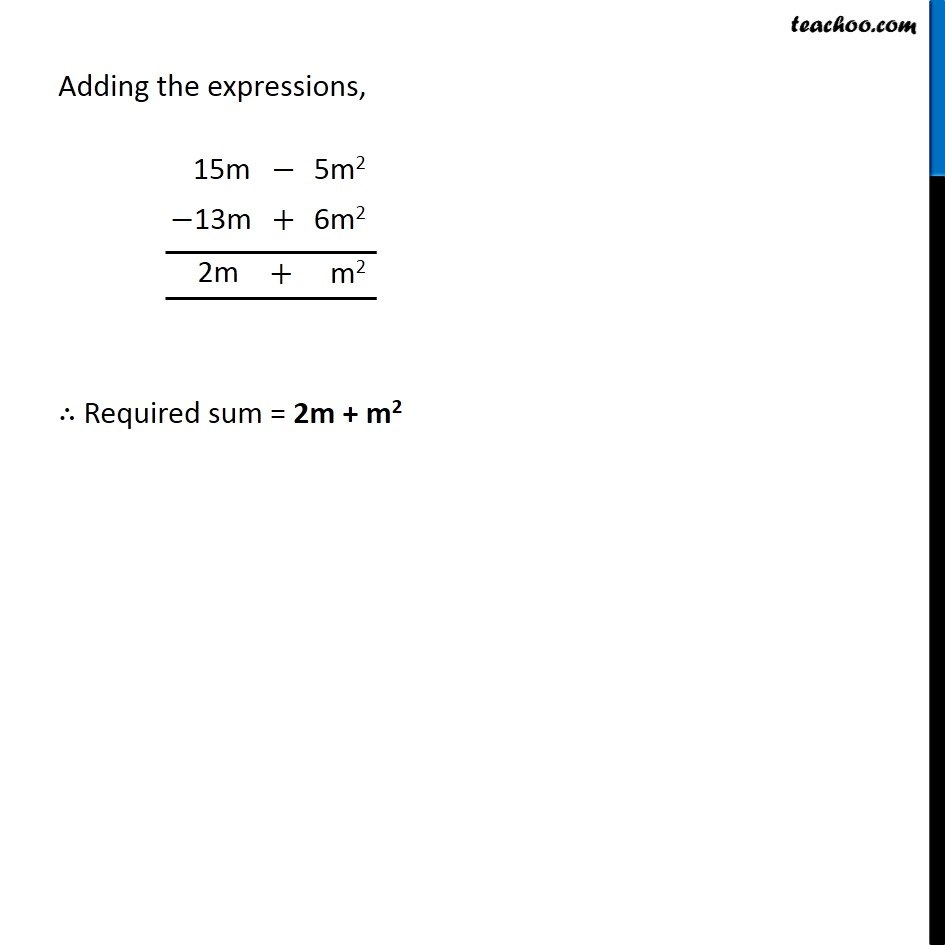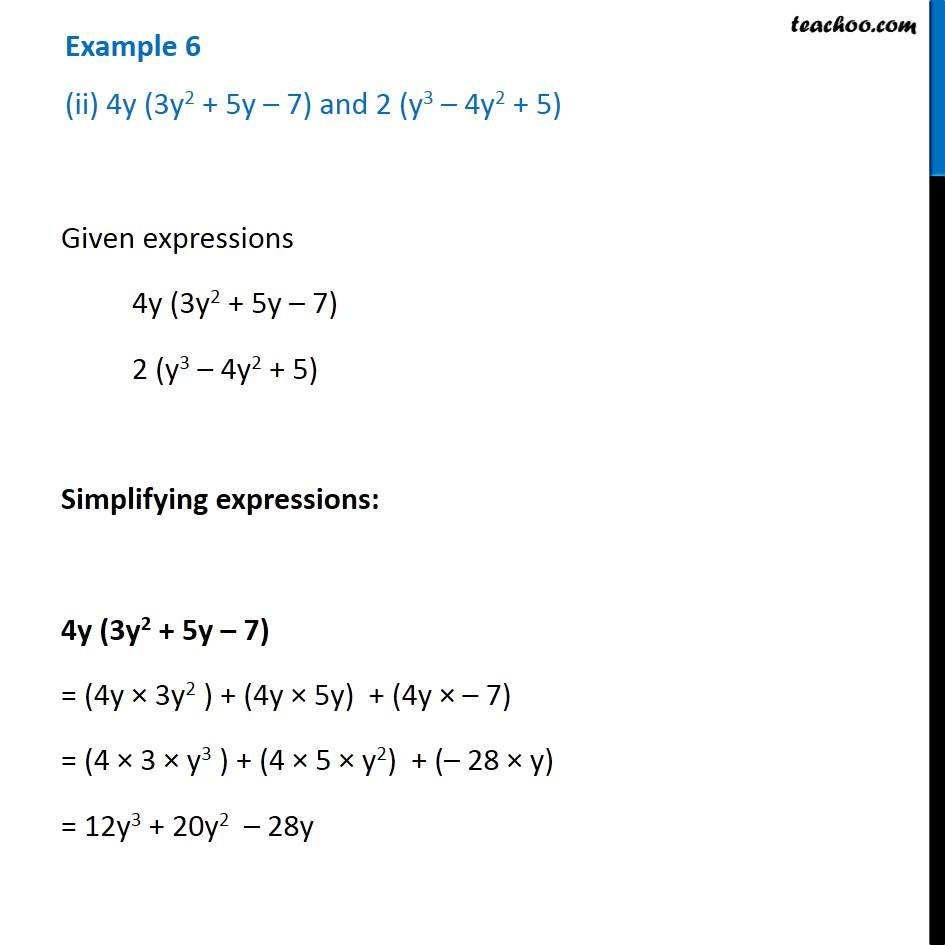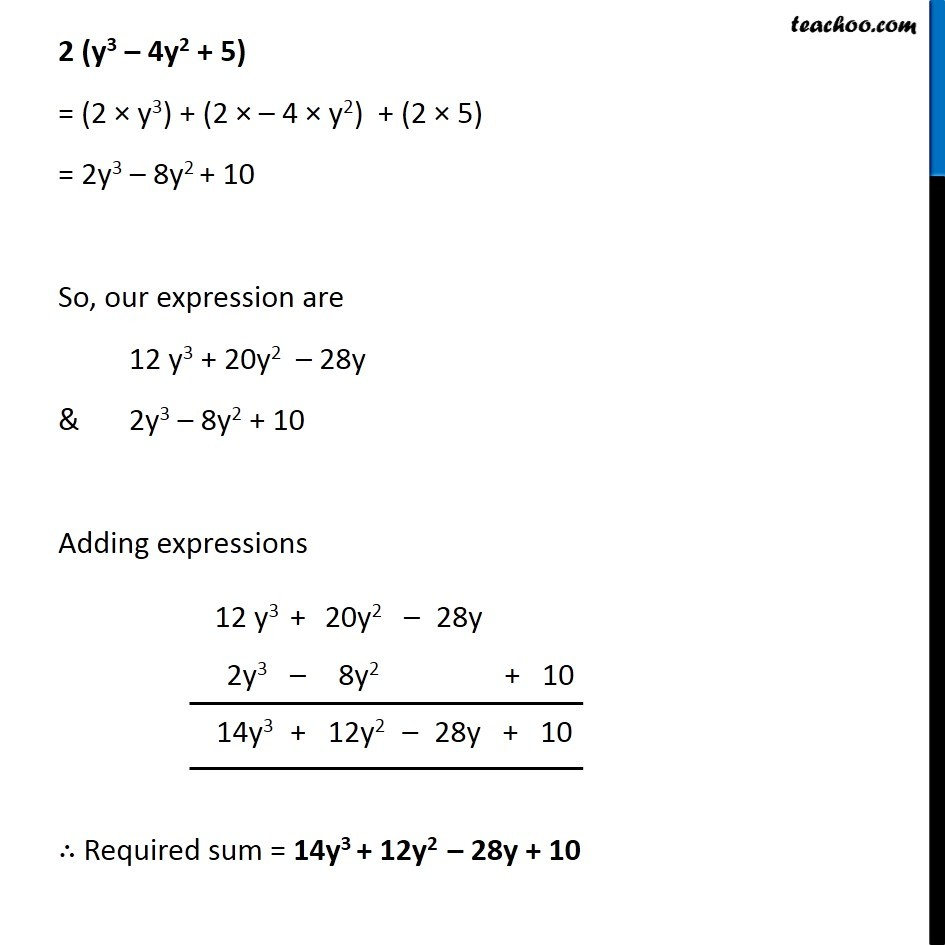1. Chapter 9 Class 8 Algebraic Expressions and Identities
2. Serial order wise
3. Examples

Transcript

Example 6 Add (i) 5m (3 – m) and 6m2 – 13m Simplifying first expression 5m (3 – m) = 5m × 3 – 5m × m = (5 × 3)m – 5(m × m) = 15m – 5m2 So our expression are 15m – 5m2 & 6m2 – 13m Adding the expressions, ∴ Required sum = 2m + m2 Example 6 (ii) 4y (3y2 + 5y – 7) and 2 (y3 – 4y2 + 5) Given expressions 4y (3y2 + 5y – 7) 2 (y3 – 4y2 + 5) Simplifying expressions: 4y (3y2 + 5y – 7) = (4y × 3y2 ) + (4y × 5y) + (4y × – 7) = (4 × 3 × y3 ) + (4 × 5 × y2) + (– 28 × y) = 12y3 + 20y2 – 28y 2 (y3 – 4y2 + 5) = (2 × y3) + (2 × – 4 × y2) + (2 × 5) = 2y3 – 8y2 + 10 So, our expression are 12 y3 + 20y2 – 28y & 2y3 – 8y2 + 10 Adding expressions ∴ Required sum = 14y3 + 12y2 – 28y + 10

Examples

About the AuthorDavneet Singh
Davneet Singh is a graduate from Indian Institute of Technology, Kanpur. He has been teaching from the past 10 years. He provides courses for Maths and Science at Teachoo.